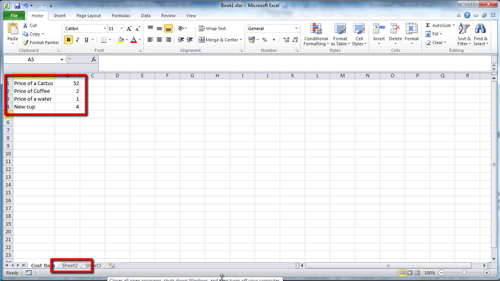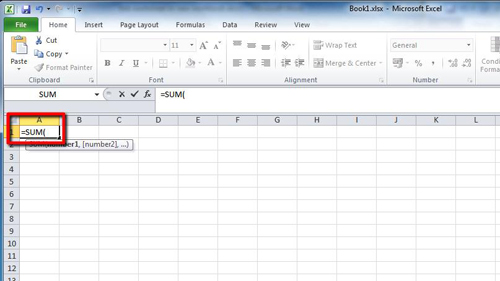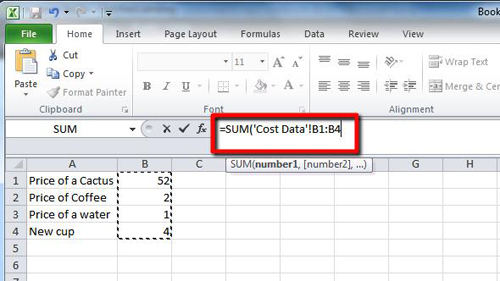## How to Link Excel 2010 formulas between worksheets

Each Excel workbook has the option to create several worksheets, this tutorial will show you how to link excel formulas between worksheets.

Step # 1 – Load the Data and Navigate to a Different Sheet

Start Excel with a sheet that has some basic information in it. Here we have a short table containing information about some items and their costs. We want to create a sum of this information in another sheet, therefore you need to navigate to “Sheet2” to enter the formula.Step # 2 – Entering the Formula

Select the cell where you want to enter the formula, in this case the cell is “A1”. As we are adding the figures together we want to use the SUM formula. Type “=SUM(“.Step # 3 – Selecting the Data

Now you need to navigate back to the first sheet, called “Cost Data” here, and select the values that you want to add together. In this case we are selecting the costs of various items, click and drag the mouse to select the data. Now click in the formula box and type “)” – you can see in the formula that it has chosen to select data specifically from the “Cost Data” sheet – press return. You will be automatically returned to “Sheet2” and can see that the formula in this sheet has summed the data from the first sheet and that is how to link excel formulas between worksheets.